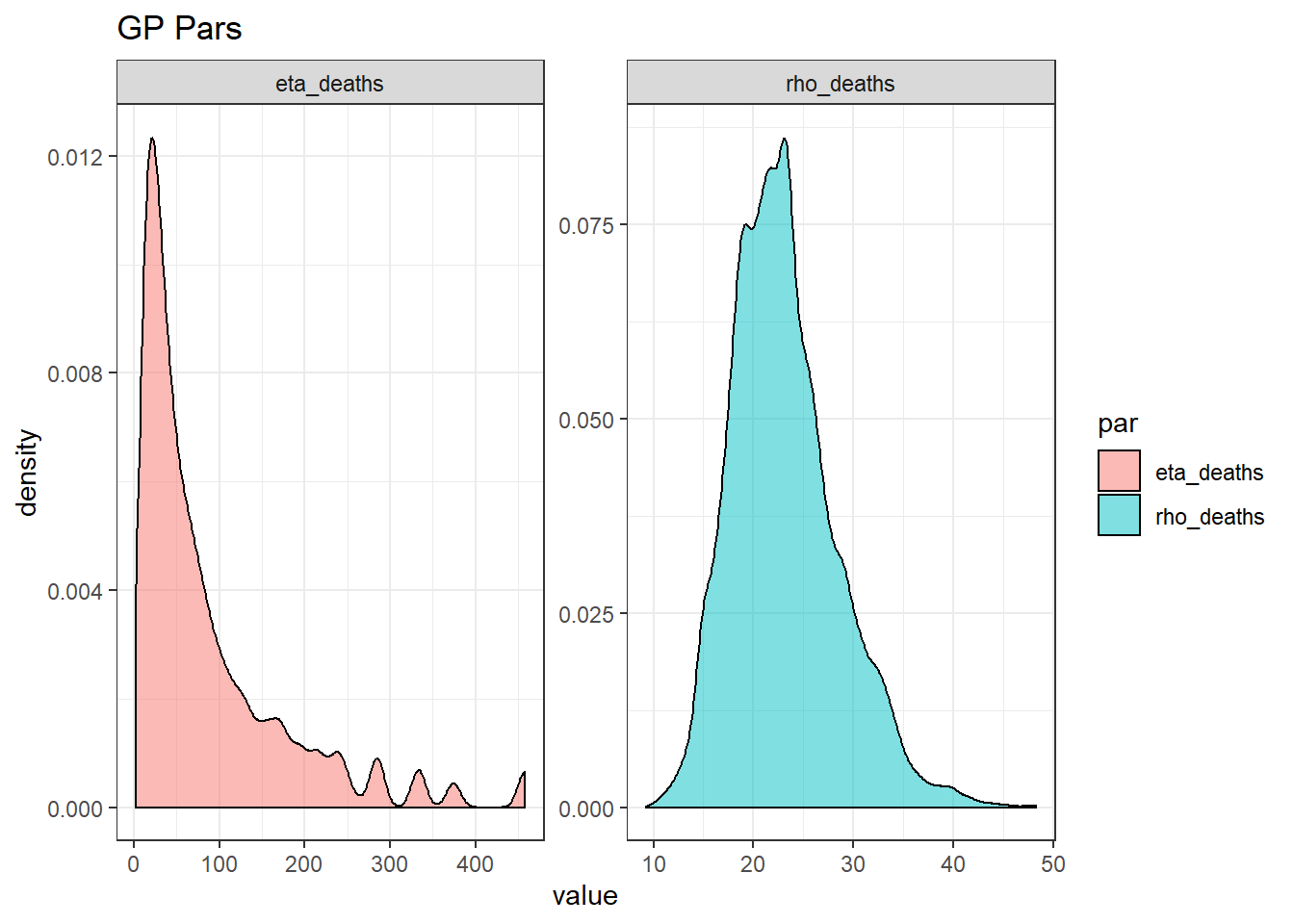# Introduction

This report is a preliminary analysis of the risk of infection (symptomatic and asymptomatic) and death (fatality rate or FR) from the corona virus. The analysis is based on the data derived from the Diamond Princess, a cruise ship which experienced an outbreak of the virus in February 2020. The passengers and crew were forced into a quarantine on the boat for a period of time. The quarantine had the unintended consequence of exposing the people to the virus and dramatically increasing the rates of infection. In total, there were 3,711 people on the boat, 619 became infected and to-date 7 have died.

The current date of this analysis is March 10, 2020.

The Diamond Princess data is unique in that all (or almost all) the passengers and crew were tested for the virus. As far as I know, this is the only meaningful data set where the denominator of the corona risk rates is known. However, there are still a significant number of passengers who have not recovered, or worse, are still in critical condition. Therefore, the numerator of the risk rates of death is only partially known. On the other hand, the risk rates of infection are known.

Warning: This analysis is a “naive” analysis, in that I do not adjust for future expected deaths. The fatality rates therefore represent a floor. I will comment on this further in the report.

All code and data can be found on Github.

# Summary of Results

• The CFR for those below age 60 is very low. The fatality rate increases from age 60 and is very high for those age 70 and above: about 1% for 70s, 8% for the 80s and 16% in the 90s. That is, mortality risk is highly skewed towards the elderly.
• The risk of infection is high across all ages. The belief that young children or young adults are not susceptible to infection is false.
• The risk of becoming symptomatic is also high across all ages.
• The risk of symptomatic and asymptomatic infection increases significantly from age 50 onwards
• Roughly half the infected population is asymptomatic.

# Methodology

Software: I used Greta and Greta GP to conduct a Bayesian analysis.

Models: The basic model is binomial model with a uniform prior assumption on the risk probability. For the youngest and very oldest ages there is very little data and this prior influences the results.

I developed a model combining all age groups and a model that analyzed each age group separately. For the latter, I used two types of models: 1. The first model assumes each age category is independent from the others. 2. The second model assumes a correlation structure between each age category. This was achieved by applying a latent Gaussian Process over the age-dependent risk parameters, using a RBF (radial basis function) kernel. I tested two GP models.

2.1. The first fixed the $$\rho$$ parameter of the RBF at 10 years. That is, the correlation between age x and x+d is $$e^{-(\frac{d}{\rho})^2}$$. So if $$d=10$$ and $$\rho=10$$, the correlation between two adjacent 10-year age buckets is $$e^{-(\frac{10}{10})^2}=0.36$$. If $$\rho=20$$, the correlation is 0.778. The tested model fixed $$\rho=10$$. 2.2. The second model, treated $$\rho$$ as a parameter. For this second model, I set the prior for $$\rho$$ to have a mean of 20 and a standard deviation of 5. The model estimated the posterior mean value of $$\rho$$ of 22 with a 95% credible interval of 14 to 35.

The GP Model under 2.2 represents an advanced model and I think gives a more accurate analysis of the fatality rates. The GP model has the advantage of smoothing out the risk rates across the age buckets and recognizing that the risk rates between age buckets is almost certainly correlated.

# Future Deaths

Based on my quick analysis, it would not surprise me if there will be additional deaths in the exposed population. Most of the infections have now occured at least 20 days ago. I would expect the fatality rates to possibly climb proportiontely by 20% to 50% (e.g. a 10% “naive” fatality rates could translate to an ultimate 12% to 15% rate), but am doubtful these ultimate fatality rates would double over the naive levels. This area still need further analysis (see Russel below for more information).

However, the analysis below is very useful in its own right, even ignoring future deaths.

# Data Sources

Note that Russel conducts a similar analysis but adjusts for outstanding deaths. My data of the age distribution differ from Russel and are based on information from Wikipedia. There are 7 deaths, 4 in the 80s, 2 in the 70s and 1 unknown. I assigned the unknown proportionately to the 80s and 70s buckets.

# Summary of Results

## A. Combined Age Category Model

### Risk Rates

The "theta" amounts represent the parameters of the binomial distribution and are the rates of infection or fatality.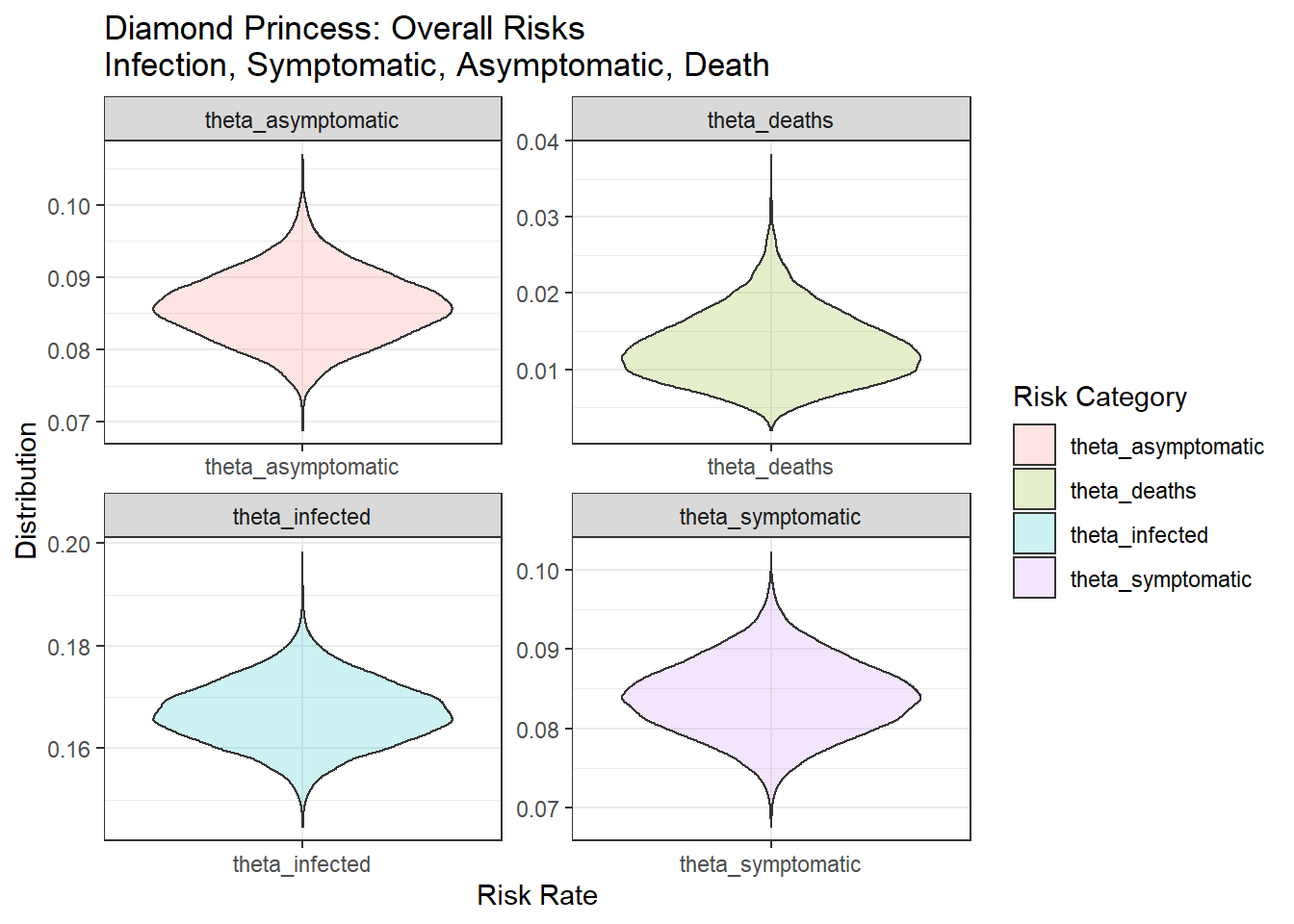## B. Independent Age Category Model

### Risk Rates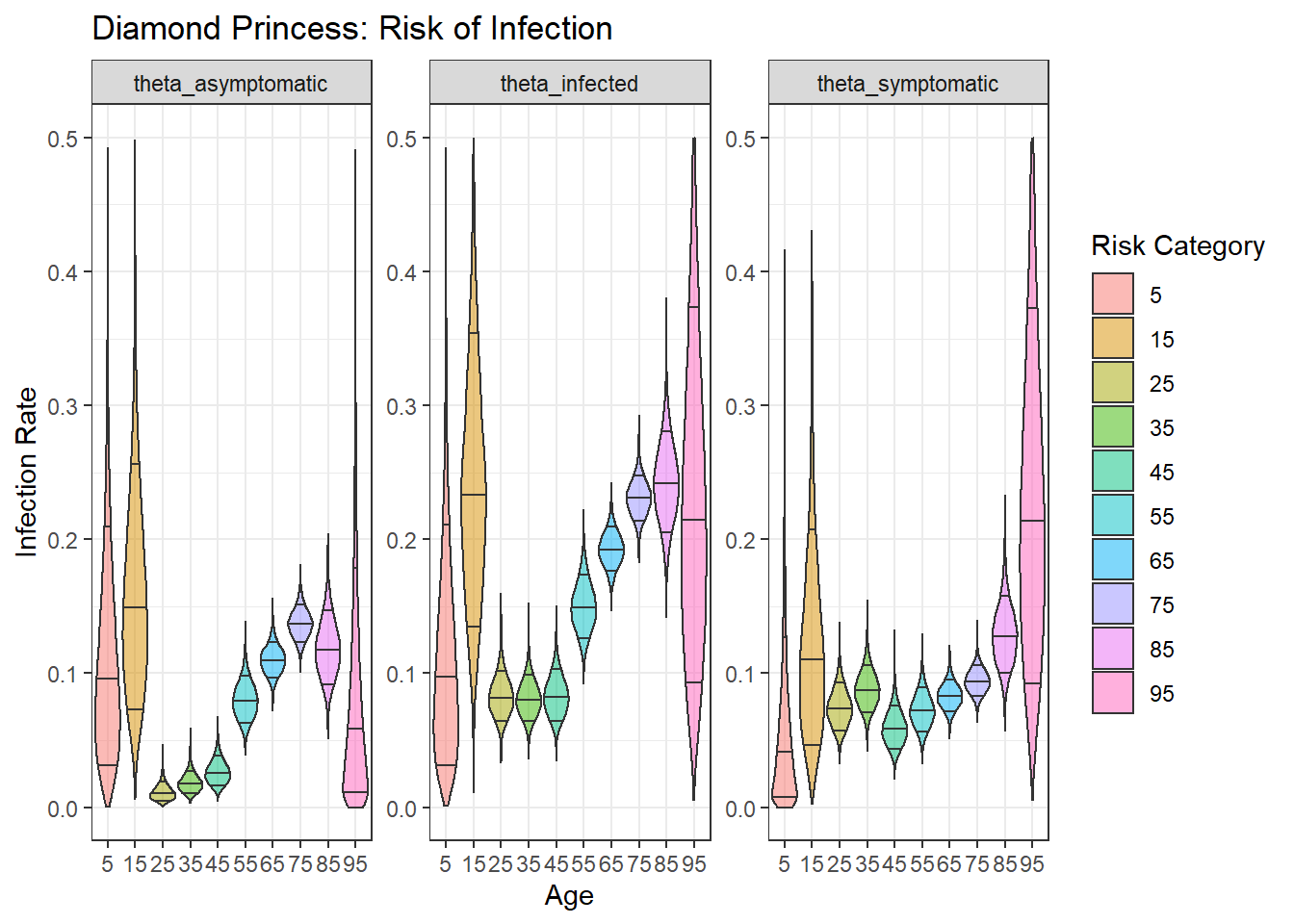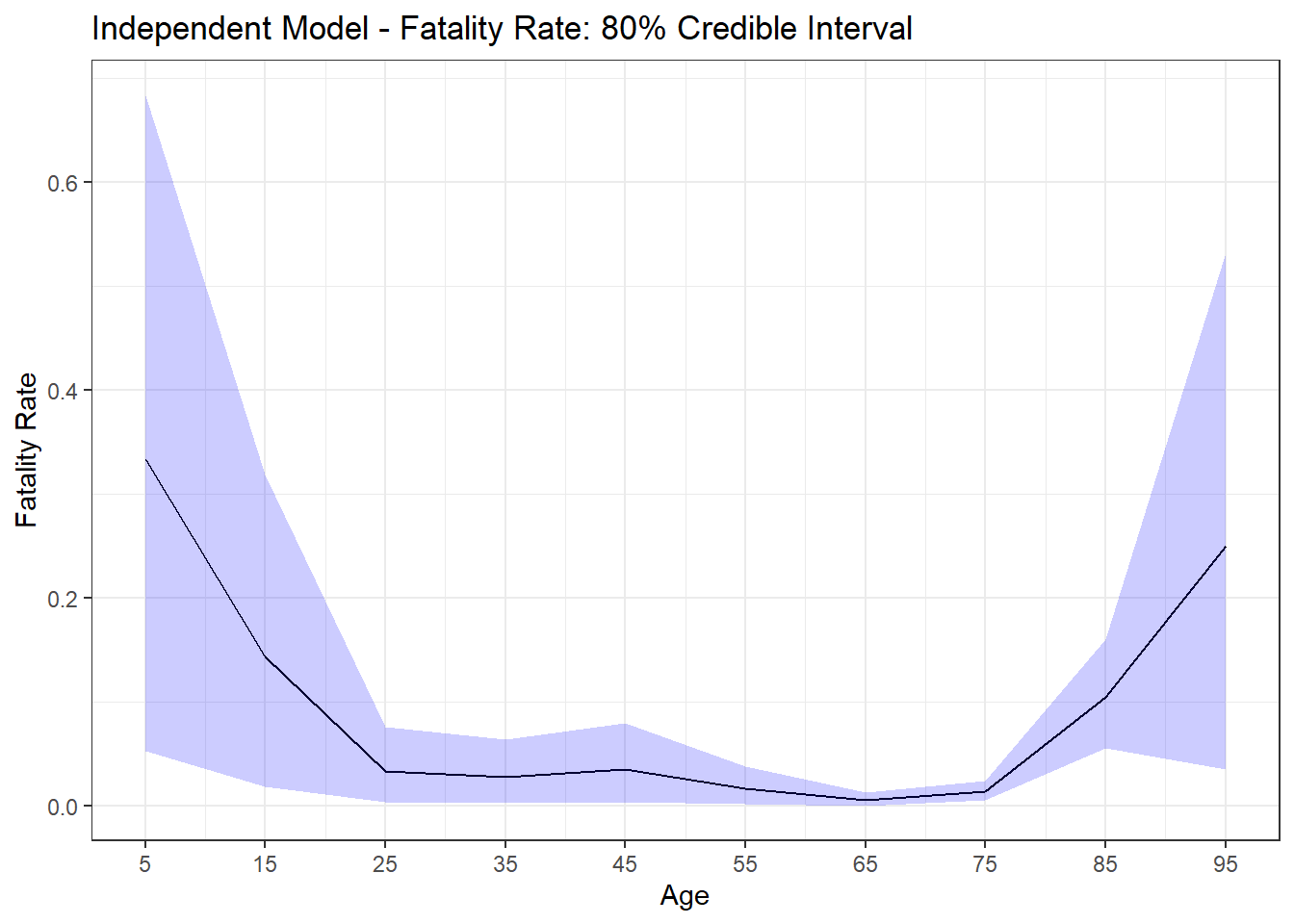Notes: There are very limited data for the age categories 5, 15 and 95. Here the uniform plays an outsize role, the resultant posterior values for these age buckets suggests a uniform prior might not be the most optimal prior, and a prior skewed towards lower risk rates might be more appropriate.

## C. Gaussian Process Model: Fixed Rho

Fixed $$\rho=10$$

### Fatality Rates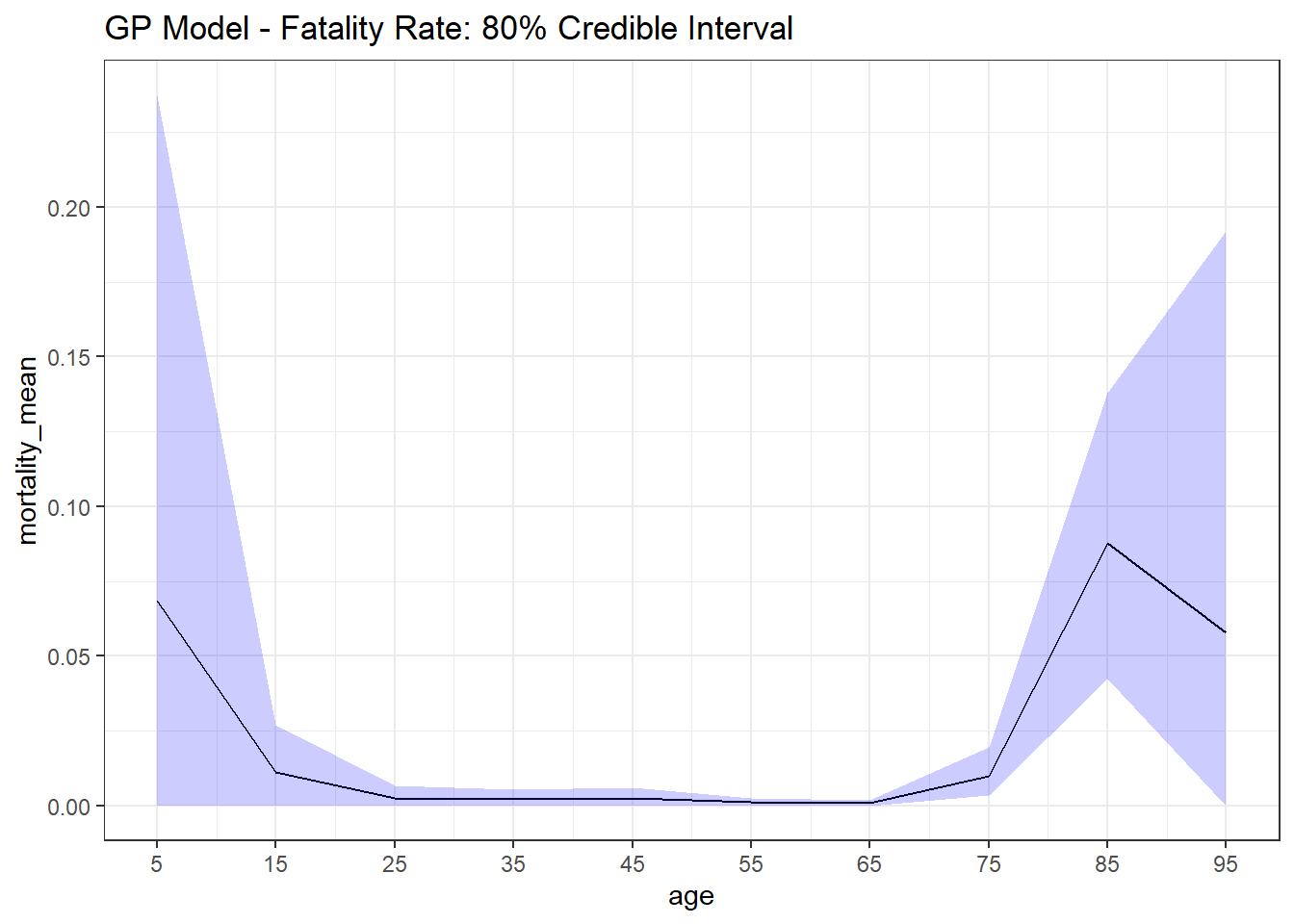## C. Gaussian Process Model: Variable Rho

Fixed $$\rho=10$$

### Fatality Rates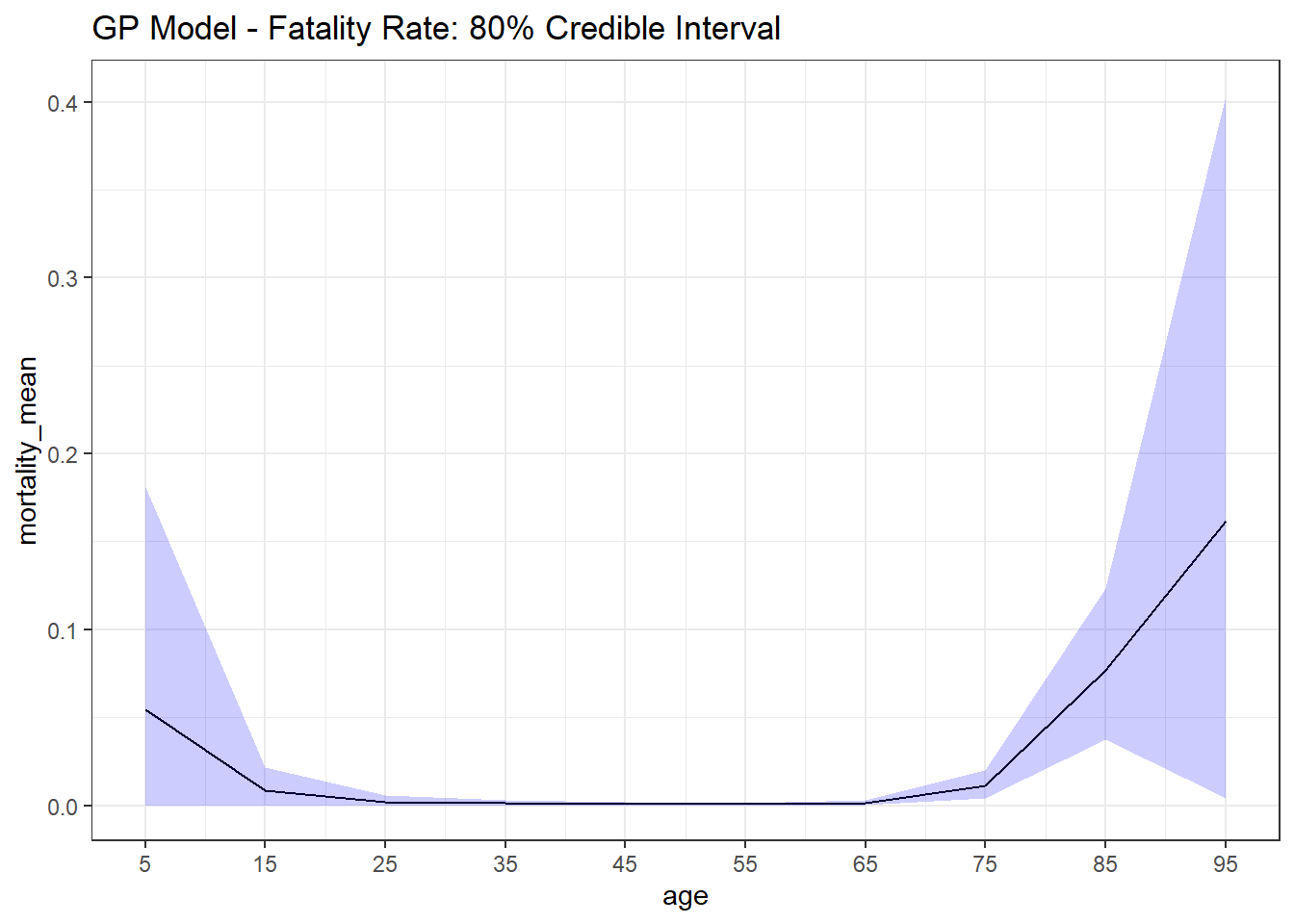### Model Parameters